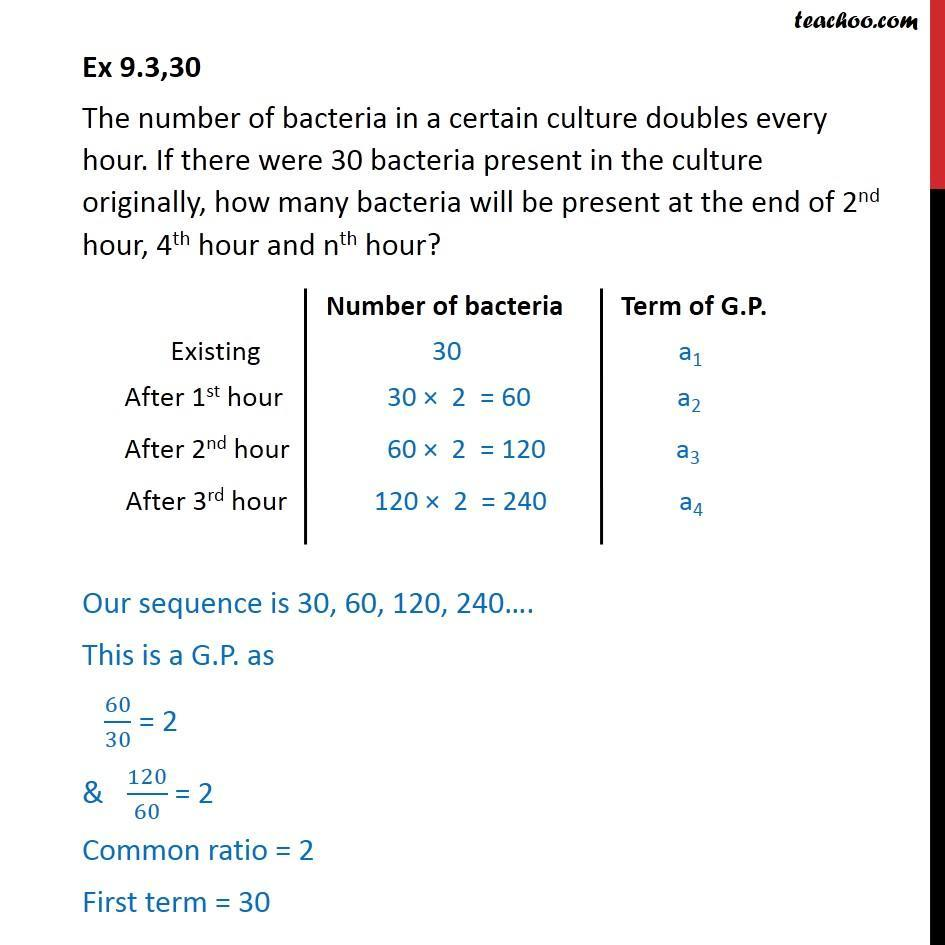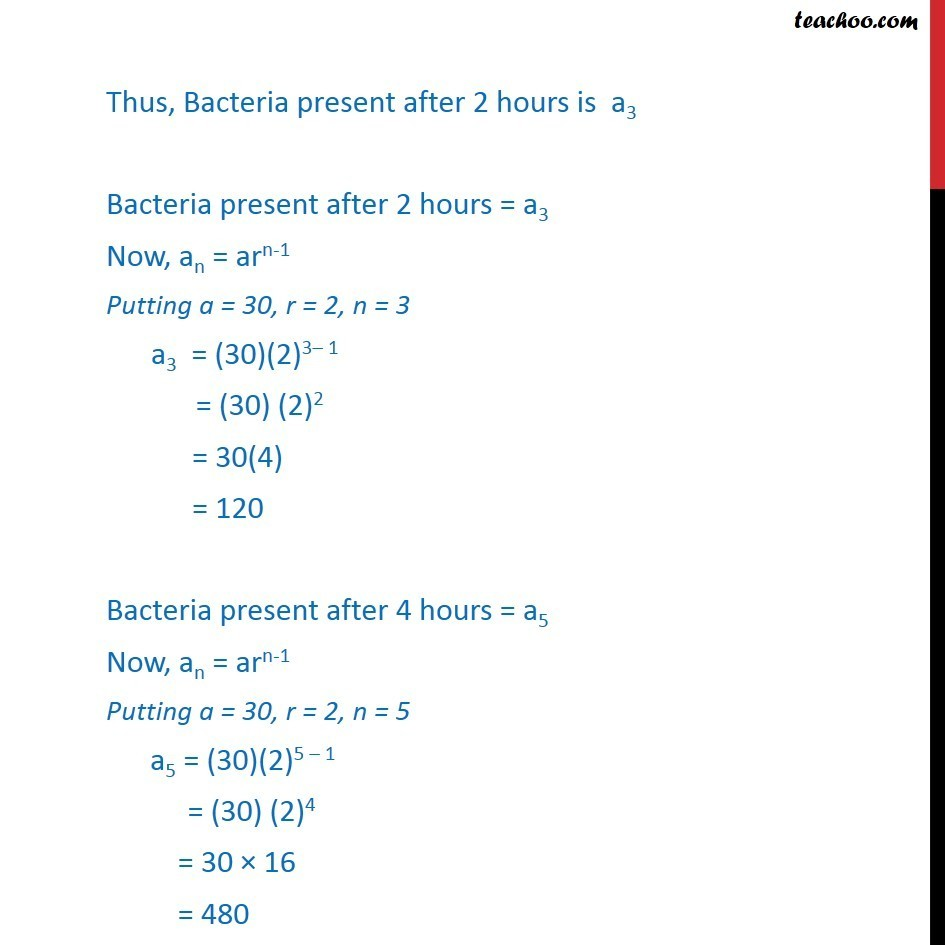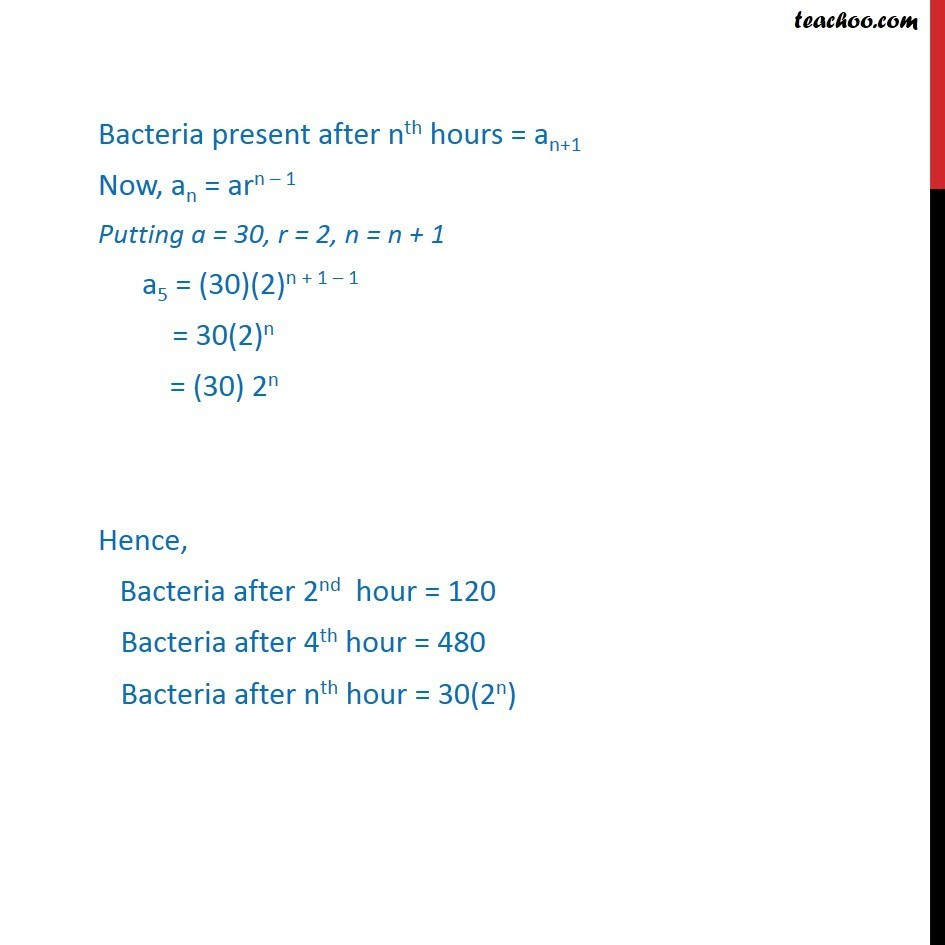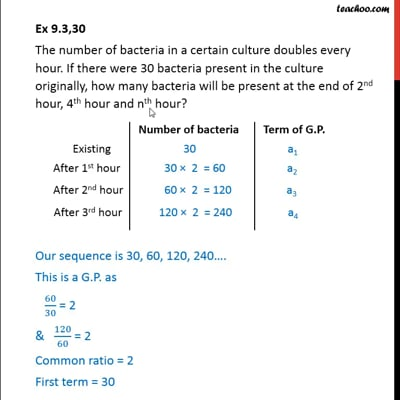Geometric Progression(GP): Statement

Chapter 9 Class 11 Sequences and Series (Term 1)
Concept wiseThis video is only available for Teachoo black users

### Transcript

Ex 9.3,30 The number of bacteria in a certain culture doubles every hour. If there were 30 bacteria present in the culture originally, how many bacteria will be present at the end of 2nd hour, 4th hour and nth hour? Our sequence is 30, 60, 120, 240 . This is a G.P. as 60/30 = 2 & 120/60 = 2 Common ratio = 2 First term = 30 Thus, Bacteria present after 2 hours is a3 Bacteria present after 2 hours = a3 Now, an = arn-1 Putting a = 30, r = 2, n = 3 a3 = (30)(2)3 1 = (30) (2)2 = 30(4) = 120 Bacteria present after 4 hours = a5 Now, an = arn-1 Putting a = 30, r = 2, n = 5 a5 = (30)(2)5 1 = (30) (2)4 = 30 16 = 480 Bacteria present after nth hours = an+1 Now, an = arn 1 Putting a = 30, r = 2, n = n + 1 a5 = (30)(2)n + 1 1 = 30(2)n = (30) 2n Hence, Bacteria after 2nd hour = 120 Bacteria after 4th hour = 480 Bacteria after nth hour = 30(2n)